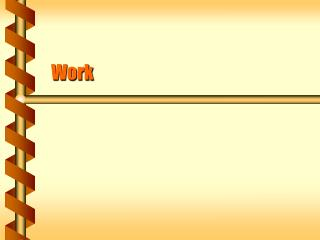DownloadDownload PresentationWork

# Work

Télécharger la présentation## Work

- - - - - - - - - - - - - - - - - - - - - - - - - - - E N D - - - - - - - - - - - - - - - - - - - - - - - - - - -
##### Presentation Transcript

1. Work

2. Hard Work? • True or false, did you do work on the object listed? • You hold a book in one hand while standing for 10 minutes. • You start a resting ball rolling across a level table. • You move a heavy box from one desk top to another of equal height. • You carry a pen up a flight of stairs.

3. Force over a Distance • In physics, work is the force acting on object over a distance. • Forces acting on objects at rest do no work.

4. Creating Motion • Work is force acting over a distance. • Changing motion requires a force: F = ma. • Force is applied over a finite distance. • Forces that change motion may do work. v = 0 F v > 0

5. Work in a Direction • The force must act in the direction of the displacement to be work. Force acts in this direction The object moves in this direction Not all the force is in the direction of motion.

6. Beginning and End • Only the force acting in the direction of displacement counts towards work. • Displacement considers the beginning and end points. • Forces acting perpendicular to an object’s motion do no work. • Linear motion with perpendicular forces • Uniform circular motion FN FN FG FG

7. Scalar Product • Finding the component of a vector in the direction of another vector is called the scalar product. A B q A|| Perpendicular vectors always have a scalar product of zero.

8. The Joule • Work is force acting over a distance, and has units equal to units of force times units of distance. • With 1-dimensional constant force, W = FDx • With vectors and a constant force, W = FDx cosq • The unit of work is the joule (J) = 1 N m.

9. Constant Force • Lifting an object requires a constant force equal to gravity, F = mg. • The work done by the lifter is W = FDx = mgh. • The work done by gravity is W = - FDx = -mgh. mg x = h -mg mg -mg

10. Individual Force • Work can be done by individual forces, or by a net force. • Constant velocity has net force of zero. • A net force of zero may include forces that are not zero. • Force acting through a distance applies to separate forces. Dx FN Ffr F Fg

11. Dissipative Force • A man is letting a 300 kg piano slide 4 m at constant velocity down a 30° incline while exerting a 400 N force on the horizontal. What work does he do? • The component of the force is (400 N)(cos 30) = -350 N • Negative since it is opposite the displacement • The work is (-350 N)(4 m) = -1400 N m = -1400 J Dx F next